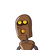# How many square tiles of side 25 cm will be required to cover the floor of a corridor which is 8 m long and 6 m broad​

How many square tiles of side 25 cm will be required to cover the floor of a corridor which is 8 m long and 6 m broad​

### 1 thought on “How many square tiles of side 25 cm will be required to cover the floor of a corridor which is 8 m long and 6 m broad​”

1.area of rectangle =l*b

given

length= 8m

8m=800cm

6m=600cm

area of rectangle=l*b

=800*600

=4,80,000

area of tiles

25*25=625sqcm

4,80,000/625=768

Therefore number of tiles required=768

Step-by-step explanation: